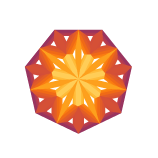12:08 AM
2A topological space $X$ is said to have the countable chain condition (ccc) if every collection of open and disjoint subsets of $X$ is at most countable. This definition can be found in L. Steen, J. Seebach: Counterexamples in Topology. p.22. I am looking for a book or an article that contain the...

1 hour later…1:26 AM
1Consider the cyclic group $C_{p^N}$ of order $p^N$, and let $k$ be a field of characteristic $p$. I would like to know what the algebra structure on the homology $H_*(C_{p^N};k)$ induced by the Pontryagin product is. The homology $H_*(C_{p^N};k)$ is a Hopf algebra that is dual to the cohomology H...

3 hours later…4:55 AM
2Let $D=\{z\in \mathbb C:|z|\leq 1\}$ be the unit disc in the complex plane, with interior $U=\{z\in \mathbb C:|z|<1\}$. Let $A\subset \mathbb C\setminus U$ be an arc intersecting $D$ only at its two endpoints $\pm i$. Let $B\subset \mathbb C\setminus U$ be an arc intersecting $D$ only at its two ...

10 hours later…3:11 PM
...

2 hours later…4:55 PM
6I just build a trait Bar with 2 functions (alpha() with implementation and beta() with only interfaces), and I hope the struct who implement Bar implements only beta(), and never implement their own alpha(). Is there any way to prevent another struct from implementing their own alpha()? trait Bar...5:25 PM
1There are several ways of describing a Hirzebruch surface, for example as the blow-up of $\mathbb{P}^2$ at one point or as $\mathbb{P}(\mathcal{O}_{\mathbb{P}^1} \oplus \mathcal{O}_{\mathbb{P}^1}(n))$. I am wondering if these surfaces admit a three-dimensional analogue and in particular if the bl...

4 hours later…9:24 PM
2The background is as follows: I consider the following differential equation $$\phi_{xx}+u\phi=\lambda \phi,\ \ \lambda=-k^2$$ where $u=u(x),\ \phi=\phi(x,\lambda)$, $\lambda$ is the spectral parameter. I designed a new solving algorithm that can numerically solve the above equation. I want to te...9:54 PM
9I'm facing a problem since this morning with webapps deployed with Apps Script that used to works fine previously. Of course no changes has been made to justify this problem. External scripts are not loaded from the HTML side, and a new error arise in the console. In order to have a reproducible ...10:37 PM
2In How to make the digits of π go around in a spiral like this? it is described how to plot pi in a spiralform (in my case as binary number): numbers = Translate[#, {-4.5, -10}] & /@ First[First[ ImportString[ ExportString[Style[#, FontSize -> 14, FontFamily -> "Arial"], ...